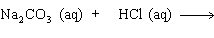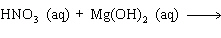Name:    Practice Test 3

Multiple Choice

1.

In an endothermic reaction,
 a) temperature of the surroundings increases. b) heat flow out of the system. c) energy is absorbed by the system. d) the products have less energy than the reactants.

2.

How many moles of CO2 can be produced by the reaction of 5.00 mol of C2H4 and 12.0 mol of O2 as shown below?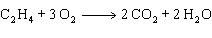a) 4.00 mol b) 10.0 mol c) 5.00 mol d) 8.00 mol

3.

How many moles of water are produced when 2.50 moles of oxygen react with excess hydrogen as shown below: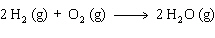a) 5.00 mol b) 3.50 mol c) 1.25 mol d) 2.00 mol

4.

In the diagrams below, the green spheres represent chlorine atoms, yellow-green spheres represent fluorine and the white spheres represent hydrogen atoms.  Based on this information, classify the reaction indicated below: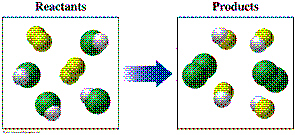a) double replacement c) Single replacement b) Decomposition d) Synthesis

5.

The reaction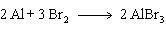is an example of
 a) decomposition b) combination c) single replacement d) double replacement

6.

How many moles of NH3 are needed to react completely with 13.6 mol of F2, as shown below?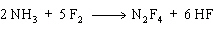a) 6.80 mol b) 5.44 mol c) 2.27 mol d) 34.0 mol

7.

How many moles of NH3 are needed to react completely with 13.6 mol of F2, as shown below?a) 2.27 mol b) 6.80 mol c) 5.44 mol d) 34.0 mol

8.

What type of reaction is shown below?

CH4  +  2 O2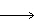CO2  +  2 H2O  +  218 kcal
 a) Single replacement b) Endothermic c) decomposition d) Exothermic

9.

Cold packs, used in athletic and medical applications, work by lowering temperature when ammonium nitrate is dissolved in water.  Which of the following statements is true for this process?
 a)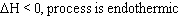b)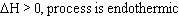c)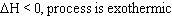d)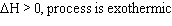10.

In the reaction shown below, how many kcal of heat is required to produce 32.0 g of O2?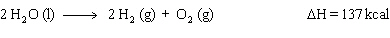a) 137 kcal b) 68.5 kcal c) 548 kcal d) 274 kcal

11.

How many grams of oxygen are needed to completely react with 200.0 g of NH3, as shown below?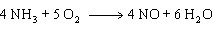a) 3.406 g b) 300.6 g c) 2.180 g d) 469.7 g

12.

When the equation shown below is balanced, the proper sequence of coefficients for each substance isa) 2, 3, 3, 1 b) 3, 2, 1, 6 c) 2, 3, 6, 1 d) 2, 3, 1, 6